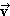# Allenaliaqi

hi

Two shuffleboard disks of equal mass, one orange and the other yellow, are involved in an elastic, glancing collision. The yellow disk is initially at rest and is struck by the orange disk moving with a speed of 5.60 m/s. After the collision, the orange disk moves along a direction that makes an angle of 36.0° with its initial direction of motion. The velocities of the two disks are perpendicular after the collision. Determine the final speed of each disk.

Two gliders are set in motion on an air track. A spring of force constant k is attached to the near side of one glider. The first glider of mass m1 has velocity1 and the second glider of mass m2 moves more slowly, with velocity2, as in Figure P9.54. When m1 collides with the spring attached to m2 and compresses the spring to its maximum compression xmax, the velocity of the gliders is. Find each of the following in terms of1,2, m1, m2 and k. (Use v_1 for1, v_2 for2, m_1 for m1, m_2 for m2 and k as necessary.)

• 8 years ago
• 60
••